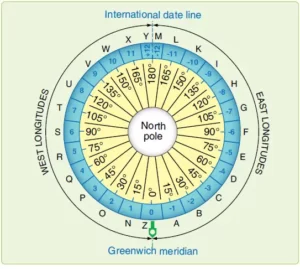# calculating time and date

### calculating time and date

PRACTICAL WORK2: CALCULATING TIME AND DATE

How to calculate the local time of a place from it longitude

This can be done using the following steps

1. Find the difference in longitude between the given longitude and the Greenwich meridian.
2. Multiply the difference in longitude by 4mins
3. Subtract or add to the GMT depending on the direction that is if it east we add and if it west we subtract.

E.G: Calculate the local time at longitude 105E and 175W when the GMT is 12:00 am.

SOLUTION

1. Longitude difference=105°-0°

=150

150*4=420MINS

420MINS/60MINS=7hours

Since longitude is the east we add; 12:00+7=7:00 pm

1. Longitude difference=175°-0°

=175

175*4=700MINS

700MINS/60MINS=11:40

Since longitude is the west we subtract; 12:00-11:40=12:20 pm

How to calculate longitude from local time

1. Work out the time difference between local time and GMT
2. Convert the time difference into longitude
3. Adjust the longitude depending on whether the time is ahead or behind the GMT

E.G: What is the longitude of Cairo whose local time is 4:00 pm when GMT is 2:00 pm?

SOLUTION

Time difference=4-2=2hours

2*60=120MINS

120MINS/4MINS=30°

Since the time of Cairo is ahead of the GMT it implies it is EAST

Longitude of Cairo= 30°E